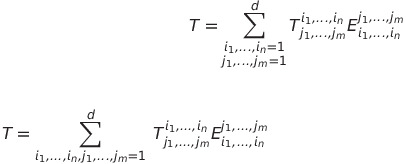# Testing latex

## Recommended Posts

$\frac{\Delta y}{\Delta x} = \frac{f(x+h)- f(x)}{h}$

$\Delta y$

$y = \int f(x) dx$

Edited by John2020
##### Share on other sites

Some further tests:

$\sum \vec{F}_{T} = \frac{\sum \vec{\tau}_{T}}{|\left(\vec{r}_{A} - \vec{r}_{R} \right)|}$

Edited by John2020
##### Share on other sites

Some further tests:

Edited by John2020
##### Share on other sites

• 2 weeks later...

Testing relativity formulas for the thread which name we do not mention...

$t = \frac{{t}' + \frac{v{x}'}{c^{2}}}{\sqrt{1 - \frac{v^{2}}{c^{2}}}}$

$t = \frac{{0} + {0.8} . {0.6} }{\sqrt{1 - {0.8 ^{2}}}}$

Edited by Eise
##### Share on other sites

• 5 months later...

Testing more stuff.

$f\left(x\right)=\begin{cases} 1, & x\in\mathbb{Q}\\ -1, & x\in\mathbb{R}-\mathbb{Q} \end{cases}$

Testing more stuff.

$\textrm{int}C\neq\textrm{Ø}\Rightarrow\textrm{if }x\in C\Rightarrow\in B_{\epsilon}\left(x\right)\subseteq C\Rightarrow\mu\left(C\right)\geq\mu\left(B_{\epsilon}\left(x\right)\right)>0$

Edited by joigus
##### Share on other sites

Rather:

$\textrm{int}C\neq\textrm{Ø}\Rightarrow\textrm{if }x\in C\Rightarrow B_{\epsilon}\left(x\right)\subseteq C\Rightarrow\mu\left(C\right)\geq\mu\left(B_{\epsilon}\left(x\right)\right)>0$

##### Share on other sites

• 1 month later...

Total energy:

$E=\frac{mc^{2}}{\sqrt{1-v^{2}/c^{2}}}$

Rest energy:

$E_{0}=mc^{2}$

Kinetic energy:

$\textrm{K.E.}=\frac{mc^{2}}{\sqrt{1-v^{2}/c^{2}}}-mc^{2}$

Edited by joigus
##### Share on other sites

• 5 weeks later...

\begin{align} \text{text} \end{align}

$\color{blue}{\text{text}}$

text

$F = ma$

Edited by Orion1

...

Edited by Orion1
##### Share on other sites

$\int (f(x)+ dy/2)dx= \int f(x)dx+ \frac{1}{2}\int dydx$

$\frac{1}{2}\int dydx$

Inline: $$dy\left( x \right) = y'\left( x \right) dx$$.

Edited by joigus
##### Share on other sites

17 hours ago, joigus said:

(f(x)+dy/2)dx=f(x)dx+12dydx

12dydx

Inline: dy(x)=y(x)dx .

@joigus, how did you center your equations?

I cannot get \begin{center} command to work.

Edited by Orion1
##### Share on other sites

1 hour ago, Orion1 said:

@joigus, how did you center your equations?

I cannot get \begin{center} command to work.

Edited by Orion1

...

Edited by Orion1
##### Share on other sites

• 1 year later...

${\not}R$

##### Share on other sites

• 2 months later...

Overlapping symbols test:

\mathclap{\fullmoon}\fullmoon

$\mathclap{\fullmoon}\fullmoon$

Pitty.

##### Share on other sites

Source:

$\begin{displaymath} T=\sum_{ \mathclap{\substack{i_1,\ldots,i_n=1 \\ j_1,\ldots,j_m=1}}}^d T_{j_1,\ldots,j_m}^{i_1,\ldots,i_n} E_{i_1,\ldots,i_n}^{j_1,\ldots,j_m} \end{displaymath}$

$\begin{displaymath} T=\sum_{ i_1,\ldots,i_n,j_1,\ldots,j_m=1}^d \; T_{j_1,\ldots,j_m}^{i_1,\ldots,i_n} E_{i_1,\ldots,i_n}^{j_1,\ldots,j_m} \end{displaymath$

Source:

\begin{displaymath}
T=\sum_{
i_1,\ldots,i_n,j_1,\ldots,j_m=1}^d \;
T_{j_1,\ldots,j_m}^{i_1,\ldots,i_n}
E_{i_1,\ldots,i_n}^{j_1,\ldots,j_m}
\end{displaymath}

Test result:
$\begin{displaymath} T=\sum_{ i_1,\ldots,i_n,j_1,\ldots,j_m=1}^d \; T_{j_1,\ldots,j_m}^{i_1,\ldots,i_n} E_{i_1,\ldots,i_n}^{j_1,\ldots,j_m} \end{displaymath}$

Expected result:(I got curious about @joigus test... wanted to learn)

Edited by Ghideon
trial & error
##### Share on other sites

Maybe this one works for "male" and "female"?

\male

Undefined control sequence \male

\female

Undefined control sequence \female
DDo

On 11/5/2022 at 2:35 PM, Ghideon said:

Source:

$\begin{displaymath} T=\sum_{ \mathclap{\substack{i_1,\ldots,i_n=1 \\ j_1,\ldots,j_m=1}}}^d T_{j_1,\ldots,j_m}^{i_1,\ldots,i_n} E_{i_1,\ldots,i_n}^{j_1,\ldots,j_m} \end{displaymath}$

Unknown environment 'displaymath'

Source:

\begin{displaymath}
T=\sum_{
i_1,\ldots,i_n,j_1,\ldots,j_m=1}^d \;
T_{j_1,\ldots,j_m}^{i_1,\ldots,i_n}
E_{i_1,\ldots,i_n}^{j_1,\ldots,j_m}
\end{displaymath}

Test result:
Unknown environment 'displaymath'

Expected result:

(I got curious about @joigus test... wanted to learn)

I think you don't need environment "displaymath" for that one. Simply:

$T=\sum_{\begin{array}{c} i_{1},\cdots,i_{n}=1\\ j_{1},\cdots,j_{m}=1 \end{array}}^{d}T_{i_{1}\cdots i_{n}}^{j_{1}\cdots j_{m}}E_{j_{1}\cdots j_{m}}^{i_{1}\cdots i_{n}}$

should work. Let's see:

$T=\sum_{\begin{array}{c} i_{1},\cdots,i_{n}=1\\ j_{1},\cdots,j_{m}=1 \end{array}}^{d}T_{i_{1}\cdots i_{n}}^{j_{1}\cdots j_{m}}E_{j_{1}\cdots j_{m}}^{i_{1}\cdots i_{n}}$

##### Share on other sites

• 4 weeks later...

$\frac{1}{2}$

Edited by Mordred
##### Share on other sites

$\array{ \mathfrak{g} \times X && \overset{R}{\longrightarrow} && T X \\ & {\llap{pr_2}}\searrow && \swarrow_{\rlap{p}} \\ && X }$

${\small\begin{array}{|c|c|c|c|c|c|c|c|c|c|}\hline Particle& Spin & g & Q &B&L_e &L_\mu&M (Mev)&\tau\\\hline \gamma&1&2&0&0&0&0&<3*10^{-33}&stable\\\hline e^-&1/2&2&-1&0&1&0&0.511&>2*10^{22}yrs\\\hline e+&1/2&2&1&0&-1&0&0.511&>2*10^{22}yrs\\\hline v_e&1/2&1&0&0&1&0&,5*10^{-5}&stable\\\hline \overline{v}_e&1/2&1&0&0&-1&0&<5*10^{-5}&stable\\\hline \mu^-&1/2&2&-1&0&0&1&105.7&2.2*10^{-6}sec\\\hline \mu^+&1/2&2&1&0&0&-1&105.7&2.2*10^{-5}sec\\\hline v_\mu&1/2&1&0&0&0&1&<0.25&>10^{32}yrs\\\hline \overline{v}_\mu&1/2&1&0&0&0&-1&<0.25&>10^{32}yrs \\\hline p&1/2&2&1&1&0&0&938.3&.10^{32}yrs\\\hline \overline{p}&1/2&2&-1&-1&0&0&938.3&>10^{32}yrs\\\hline n&1/2&2&0&1&0&0&939.6&898 sec\\\hline \overline{n}&1/2&2&&*-1&0&0&939.6&898 sec\\\hline \pi^0+&0&1&1&0&0&0&139.6&1.39*10^{-8}sec\\\hline \pi^-&0&1&0&0&0&0&135.0&8.7*10^{-17}sec\\\hline \pi^+&0&1&-1&0&0&0&139.6&2.6*10^{-8}sec\\\hline\end{array}}$

Edited by Mordred
##### Share on other sites

• 2 months later...

$\overset{m^{2}c^{4}}{\overbrace{E^{2}-c^{2}\boldsymbol{p}^{2}}}+\overset{0}{\overbrace{\hbar^{2}\omega^{2}-c^{2}\hbar^{2}\boldsymbol{k}^{2}}}+2\hbar E\omega-2c^{2}\hbar\boldsymbol{p}\cdot\boldsymbol{k}=m^{2}c^{4}$

OK then.

## Create an account

Register a new account